Bulbs, batteries and meters

Voltage and current can be quantified using meters.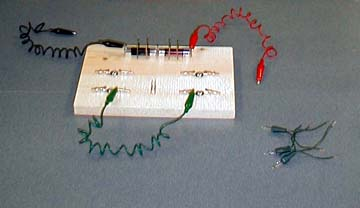A pine board with a battery holder made of nails and alligator clips is used to hold Christmas tree lights.

Material

• Christmas tree bulbs from series chains of 50 bulbs. For the best prices, buy these after Christmas. (recent retail price \$2)
• 2 x 1.5 volt AA batteries
• Two meters, one to measure voltage of 3 volts one to measure current up to one amp.
• aluminum foil
• A breadboard to connect the bulbs and batteries
• or a battery holder and alligator clips.

Assembly

Cut the lights apart leaving 10 cm (3") of wire connected to each bulb.
Cut the plastic insulation off the last 1 cm of wire, 0.5 inch.
Build a breadboard by screwing alligator clips onto a wooden plank see above.
Add a battery holder by driving nails into the plank.

To Do and Notice

Notice that the voltmeter and ammeter should both have a red lead attached to their positive terminals and a black lead attached to their COM or common terminals.

Symbols

We'll use the symbol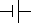for a battery, where the large plate indicates the positive end,

and the symbol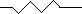for a bulb or resistor.

Connect the bulb to one battery.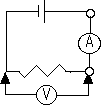Attach the voltmeter across the bulb.

Break the circuit and insert the ammeter between the battery and the bulb.

Notice that the voltmeter reads the voltage, probably near 1.5 volts.
The voltage is positive if the red lead of the voltmeter is at the higher voltage. If the red lead is attached near the positive end of the battery it will read a positive voltage. If the leads are reversed the voltage will read negative.

Notice that if positive current flows into the red lead of the ammeter that the current will be positive. Reverse the leads and the current is negative.

Here is a meter measuring the voltage across one bulb.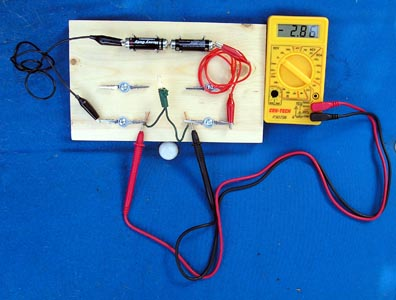A digital meter measures the voltage across one bulb (two batteries in series)

Here is a meter measuring the current through one bulb.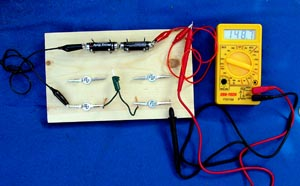Here is a meter inserted into the cicuit to measure the current through a bulb.

Build the two bulb series and parallel circuits. Measure the currents through and voltages across the bulbs in these circuits.

What's Going On?

In the series circuit with two bulbs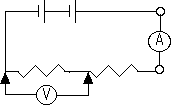Measure the voltage across one of the two bulbs.
Measure the current in the circuit.

The current through the circuit is the same everywhere.

The voltage splits between the two bulbs.
If the bulbs are identical the voltage splits equally between them, measure them both.

In the circuit with two bulbs in parallel: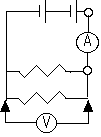Measure the voltage across a bulb and the total current delivered by the battery.Measure the current through one bulb.

The current is split between the two bulbs.
If the bulbs are identical half the current goes through each one.

Math Root

Two resistors in series act as if they were one resistor with the sum of the resistances.

R = R1 + R2

So two bulbs in series have twice the resistance of one bulb.

Doubling the resistance cuts the current in half according to ohms law.

I = V/R